# Chord Key Changer

## This is a virtual Strumstick Chord Changer Wheel

It will change (transpose) a group of song chords from Any Key (in the Blue section of the wheel) into their corresponding chords in Key of D (the Red section of the wheel). The song chords you may be starting with are transposed into chords you can use on the D Strumstick.
(If you have a G Strumstick, see bottom of page).

### Instructions to use Chord Changer Wheel:

Let's  Look first at this special case, where the Blue (starting key) Key is D.You will notice that the Blue chords are exactly the same as the Red Chords above them, and the minor chords (like Bm) are the same as the corresponding minor chords above the Red Chords. This is because we are comparing key of D (Blue) to Key of D (Red).

As we might expect, the wheel shows that D = D, G= G, A = A, Bm = Bm, etc.

Now look at this blue Key of A position next: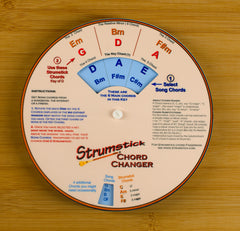Here we see that the Blue Chords are A, D, E, F#m, etc. The wheel changes (transposes) the Key of A chords to their key of D equivalents, so:
blue A becomes red DA = D),
E
= A,
D = G,
F#m = Bm
and blue Bm becomes red Em

This means if the song chords were:
A   A    D   D   F#m
F#m  E   E   Bm   Bm   E   E    A   D   A

they would become, in Key of D,
D   D     G   G   Bm   Bm    A   A   Em   Em   A   A    D   G   D

Here are two audio examples, of Key of A and then Key of D on Guitar

Key of A to Key of D (all on Guitar)

Instructions to Use:
(instructions going here)
Key of A (or F# minor)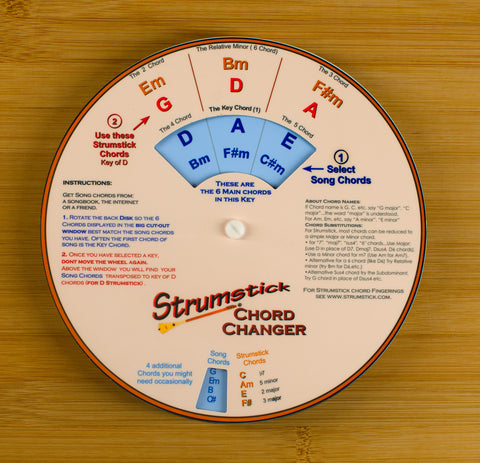Key of Bb  (or G minor)Key of B   (or Ab minor)Key of C  (or A minor)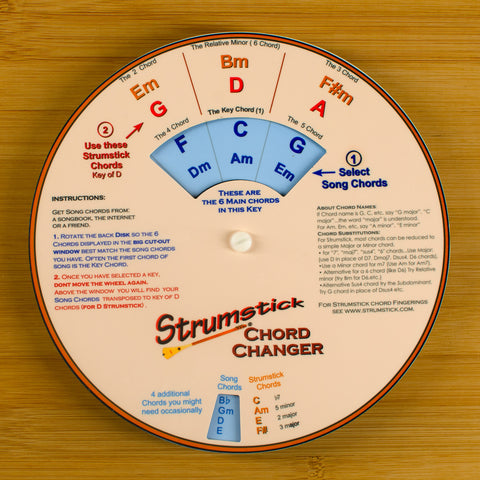Key of C#  (or Bb minor)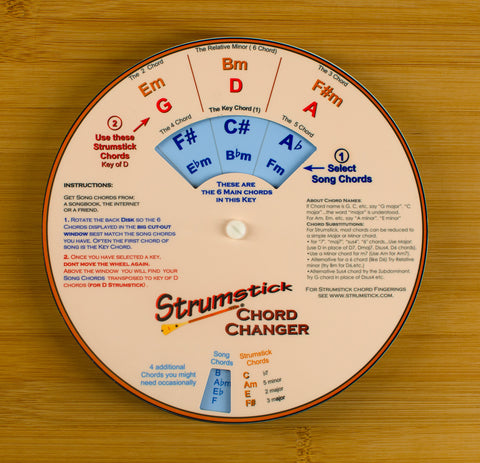Key of D  (or B minor)Key of Eb  (or C minor)Key of E (or C# minor)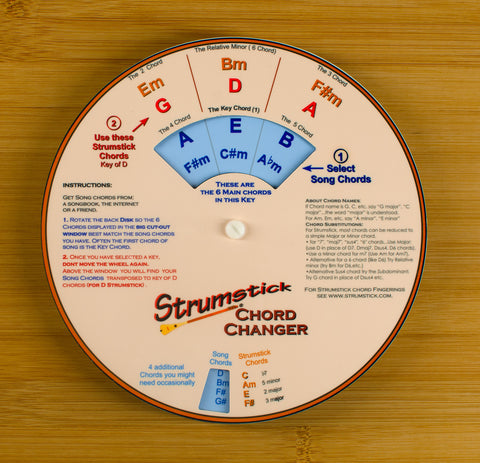Key of F (or D minor)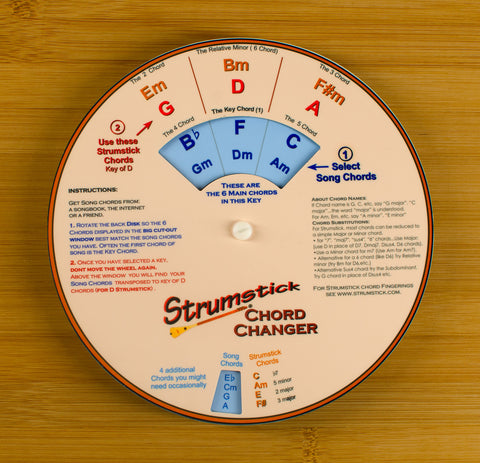Key of F# (or Eb minor)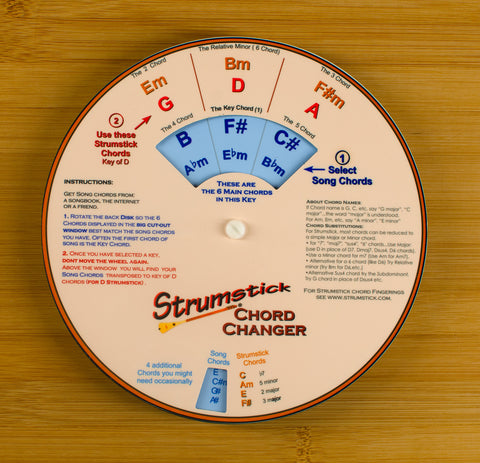Key of G (or E minor)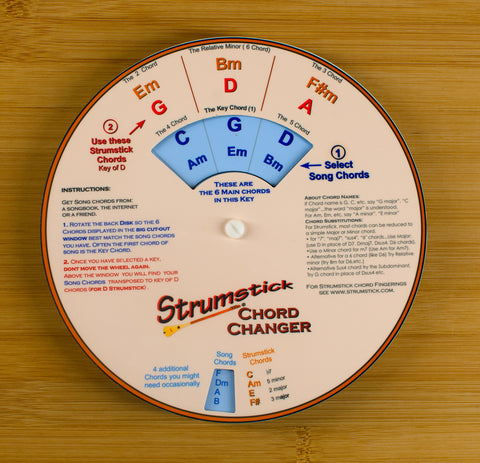Key of Ab (or F minor)